Courses

# Test: Time Response

## 20 Questions MCQ Test RRB JE for Electronics & Communication Engineering | Test: Time Response

Description
This mock test of Test: Time Response for Electronics and Communication Engineering (ECE) helps you for every Electronics and Communication Engineering (ECE) entrance exam. This contains 20 Multiple Choice Questions for Electronics and Communication Engineering (ECE) Test: Time Response (mcq) to study with solutions a complete question bank. The solved questions answers in this Test: Time Response quiz give you a good mix of easy questions and tough questions. Electronics and Communication Engineering (ECE) students definitely take this Test: Time Response exercise for a better result in the exam. You can find other Test: Time Response extra questions, long questions & short questions for Electronics and Communication Engineering (ECE) on EduRev as well by searching above.
QUESTION: 1

### The open-loop transfer function of a ufb control system is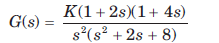The position, velocity and acceleration error constants are respectively

Solution: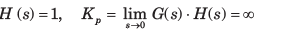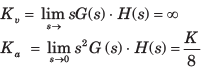QUESTION: 2

### The open-loop transfer function of a unit feedback system is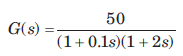The position, velocity and acceleration errorconstants are respectively

Solution: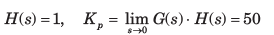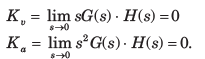QUESTION: 3

### If a type 0 system is subjected to step input, what is its effect on steady state error?

Solution:
QUESTION: 4

The forward-path transfer function of a unity feedback system is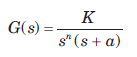The system has 10% overshoot and velocity error constant Kv =100.

The value of a is

Solution: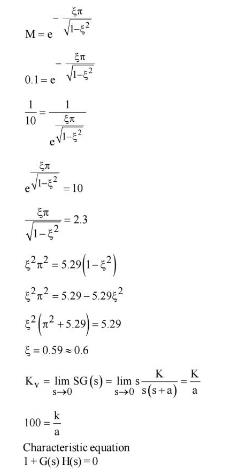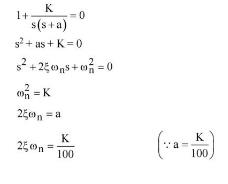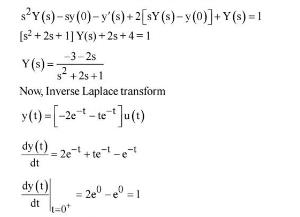QUESTION: 5

For the system shown in fig.the steady state error component due to unit step disturbance is 0.000012 and steady state error component due to unit ramp input is 0.003. The values of K1 and K2 are respectively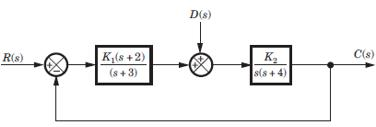Solution:

If R (s) = 0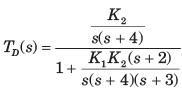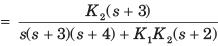Error in output due to disturbance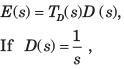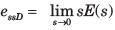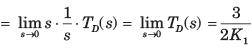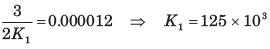Error due to ramp input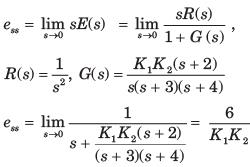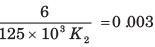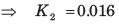QUESTION: 6

The transfer function for a single loop nonunity feedback control system is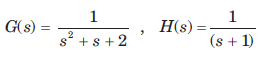The steady state error due to unit step input is

Solution:

E(s) = R(s) - C(s) H(s)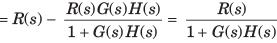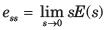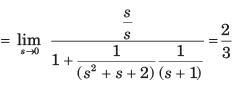QUESTION: 7

For the system of fig. the total steady state error due to a unit step input and a unit step disturbance is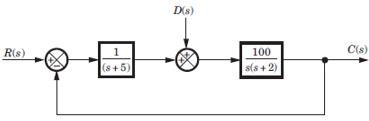Solution: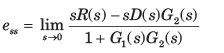where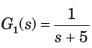and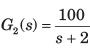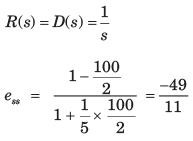QUESTION: 8

The forward path transfer function of a ufb system is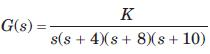If a unit ramp is applied, the minimum possiblesteady-state error is

Solution:

Using Routh-Hurwitz Criterion, system is stable for 0 < K < 2000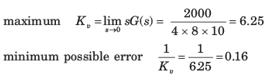QUESTION: 9

The forward-path transfer function of a ufb system is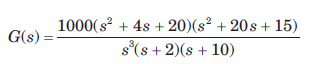The system has r(t) = t3 applied to its input. Thesteady state error is

Solution: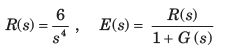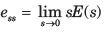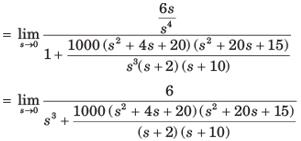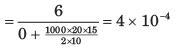QUESTION: 10

Consider a unity feedback system with forward transfer function given by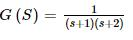The steady-state error in the output of the system for a unit-step input is _________ (up to 2 decimal places).

Solution: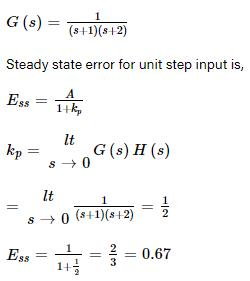QUESTION: 11

A system has position error constant Kp  = 3. The steady state error for input of 8tu(t) is

Solution:

System is zero type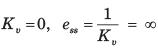QUESTION: 12

The forward path transfer function of a unity feedback system is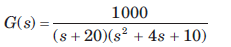For input of 60u(t) steady state error is

Solution: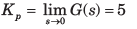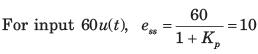QUESTION: 13

For ufb system shown in fig. the transfer function is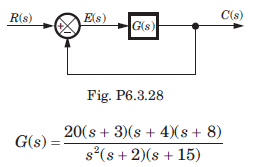If input is 30t2 , then steady state error is

Solution: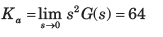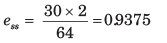QUESTION: 14

The forward-path transfer function of a ufb control system is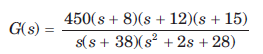The steady state errors for the test input 37tu(t) is

Solution: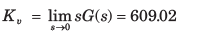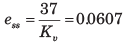QUESTION: 15

In the system shown in fig. r(t) =  1 + 2t , t > 0. The steady state error e(t) is equal to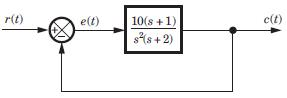Solution:

The system is type 2. Thus to step and ramp input error will be zero.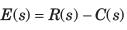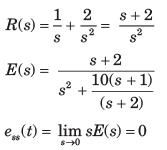QUESTION: 16

A ufb control system has a forward path transfer function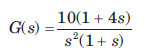If the system is subjected to an input r(t) = 1 + t + 1/2 t2 , t > 0the steady state error of the system will be

Solution:

Explanation : R(s) = (1/s)+(1/s2) + (1/s3)

E(s) = R(s) / {1 + G(s)} = 1/10s

ess = lim sE(s) = 0.1

QUESTION: 17

The system shown in fig. has steady-state error 0.1 to unit step input. The value of K is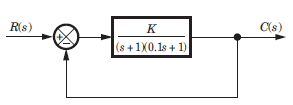Solution: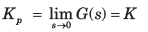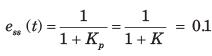QUESTION: 18

Block diagram of a position control system is shown in fig.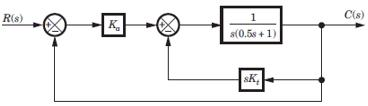If Kt = 0 and Ka= 5, then the steady state error tounit ramp input is

Solution: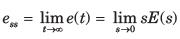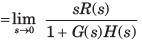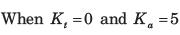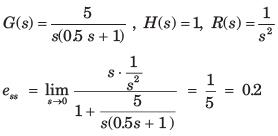QUESTION: 19

Block diagram of a position control system is shown in fig.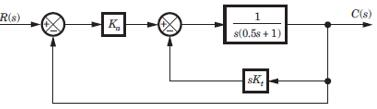If the damping ratio of the system is increased to 0.7 without affecting the steady state error, then thevalue of Ka and Kt are

Solution:

The equivalent open-loop transfer function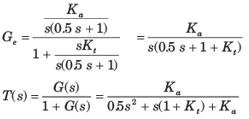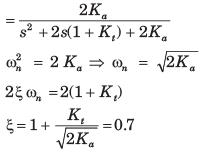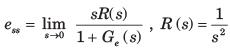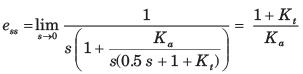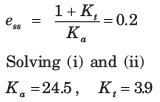QUESTION: 20

A system has the following transfer function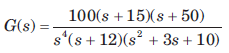The type and order of the system are respectively

Solution:

The s has power of 4 and denominator has order of 7. So Type 4 and Order 7.